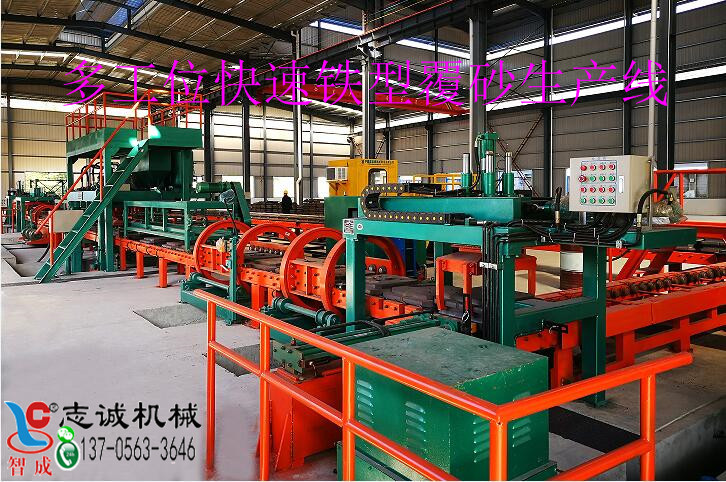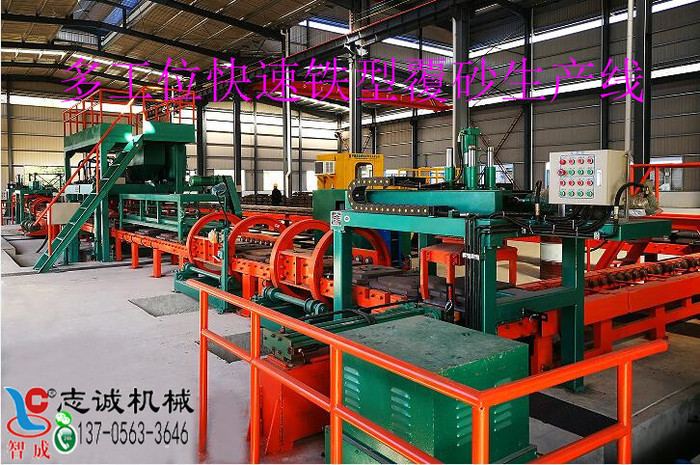# 选矿厂球磨机不同球径装球配比及实例(1)先取磨矿机全给矿样(一般分别取新给矿和返砂样，然后按比例配样)逬行筛析，分成若干粒级，如分成18〜12, 12〜10, 10〜8, 8〜6等，称重各粒级，算出各粒级质量百分比(产率)，如，，......等；

⑵用各级别粒度上限(如18、12、10、8......等)或上、下限

D10……

G重量=V有效×充填率×钢球堆密度

①按公式计算出球的总质量G:

D-球磨机筒体内径，m；

L-球磨机筒体有效长度，m;；

-介质充填率，%；

-球的堆密度，t/m3。

②依调整后的各级球比例计算出各级钢球的质量分别为GD12=G.D12,GD10=G.D10,GD8=G.D8。

⑴矿磨机全给矿筛析结果见表1

 粒级（mm） 产率（%） 粒级（mm） 产率（%） 18 〜12 18-12=20 6 〜4 6-4=5 12 〜10 12-10=40 4 〜2 4-2=4 10 〜8 10-8=15 2 〜1 2-1=4 8〜6 8-6=8 1 〜0 1-0=4

⑵按各级上限计算应配的球径并取整，见表2

 粒级（mm） 球径（mm） 粒级（mm） 球径（mm） 18 〜12 D18=120 6 〜4 D6=70 12 〜10 D12=100 4 〜2 D4=60 10 〜8 D10=90 2 〜1 D2=50 8〜6 D8=80 1 〜0 D1=40

⑶各种球的质量百分比见表3

 球径（mm） 质量比列（%） 球径（mm） 质量比列（%） 120 20 70 5 100 40 60 4 90 15 50 4 80 8 40 4

 球径（mm） 质量比列（%） 球径（mm） 质量比列（%） 120 30 80 13 100 45 60 12

⑷计算加入球的总质量G:

⑸计算各种球分别应加入质量

G120=G.120=11.928×0.3=3.85（t）

G100=G.100=11.928×0.45=5.37（t）

G80=G.80=11.928×0.13=1.55(t）

G60=G.60=11.928×0.12=1.43(t）